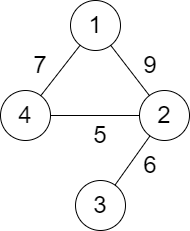Comment on page

# 2492. Minimum Score of a Path Between Two Cities

Medium
You are given a positive integer `n` representing `n` cities numbered from `1` to `n`. You are also given a 2D array `roads` where `roads[i] = [ai, bi, distancei]` indicates that there is a bidirectional road between cities `ai` and `bi` with a distance equal to `distancei`. The cities graph is not necessarily connected.
The score of a path between two cities is defined as the minimum distance of a road in this path.
Return the minimum possible score of a path between cities `1` and `n`.
Note:
• A path is a sequence of roads between two cities.
• It is allowed for a path to contain the same road multiple times, and you can visit cities `1` and `n` multiple times along the path.
• The test cases are generated such that there is at least one path between `1` and `n`.
Example 1:Input: n = 4, roads = [[1,2,9],[2,3,6],[2,4,5],[1,4,7]]
Output: 5
Explanation: The path from city 1 to 4 with the minimum score is: 1 -> 2 -> 4. The score of this path is min(9,5) = 5.
It can be shown that no other path has less score.
Example 2:Input: n = 4, roads = [[1,2,2],[1,3,4],[3,4,7]]
Output: 2
Explanation: The path from city 1 to 4 with the minimum score is: 1 -> 2 -> 1 -> 3 -> 4. The score of this path is min(2,2,4,7) = 2.
Constraints:
• `2 <= n <= 10^5`
• `1 <= roads.length <= 10^5`
• `roads[i].length == 3`
• `1 <= ai, bi <= n`
• `ai != bi`
• `1 <= distancei <= 10^4`
• There are no repeated edges.
• There is at least one path between `1` and `n`.

### 解題

func minScore(n int, roads [][]int) int {
for i:=0; i<=n; i++ {
}
}
ans := 100000000
queue := make([][]int, 0)
queue = append(queue, []int{1, 100000000})
visited := make([]bool, n+1)
visited = true
for len(queue) != 0 {
ct := queue
queue = queue[1:]
for _, a := range adj[ct] {
ans = min(ans, a)
if !visited[a] {
visited[a] = true
queue = append(queue, []int{ a, a})
}
}
}
return ans
}
func min(a, b int) int {
if a < b { return a }
return b
}
Union-find
func minScore(n int, roads [][]int) int {
group := make([]int, n+1)
minpath := make([]int, n+1)
var find func(int) int
find = func(a int) int {
if a == group[a] { return a }
return find(group[a])
}
var union func(int, int, int)
union = func(a int, b int, dis int) {
aroot := find(a)
broot := find(b)
if broot < aroot {
group[broot] = aroot
minpath[aroot] = min(dis, min(minpath[aroot], minpath[broot]))
} else {
group[aroot] = broot
minpath[broot] = min(dis, min(minpath[broot], minpath[aroot]))
}
}
for i:=0; i<=n; i++ {
group[i] = i
minpath[i] = 10000000
}